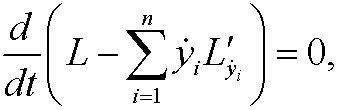3. The Hamiltonian.

According to Lagrange’s equations we have thatNow suppose that L is independent of t, that is, Lt=0. Then we can use a variant of Beltrami’s identitet (see below for derivation) and rewrite the equations aswhich by integration becomeswhere C is a constant. This is called a conservation law and the quantityis called the Hamiltonian of the system. It normally represents the total energy of the system. The analysis above thus means that if L is time independent then the totla energy of the system is preserved.

Derivation:since